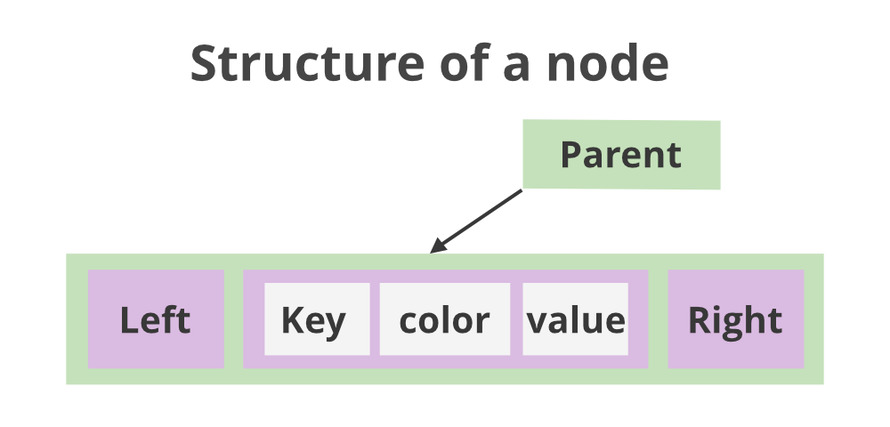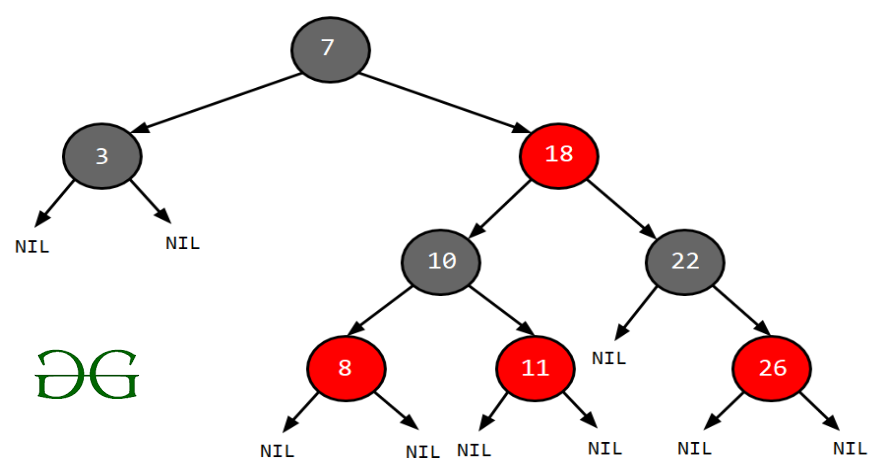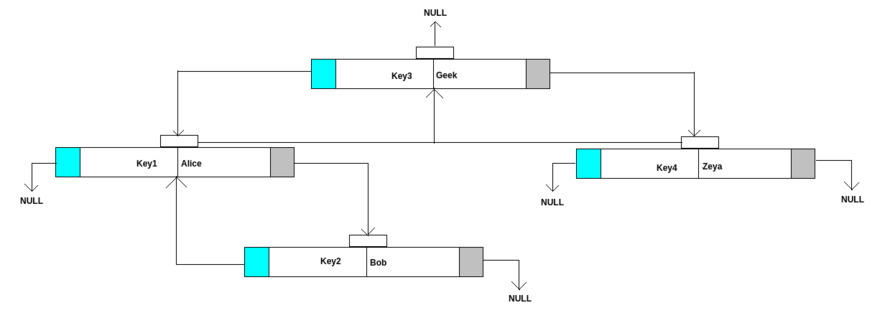Related Articles

# Internal Working of TreeMap in Java

• Difficulty Level : Easy
• Last Updated : 05 Jul, 2021

TreeMap class is like HashMap. TreeMap stores key-value pairs. The main difference is that TreeMap sorts the key in ascending order. TreeMap is sorted as the ordering of its keys, or by a Comparator provided at map creation time, depending on which constructor is used.Pre-requisite:

Let’s go through pre-requisite that primarily includes constructors available in case of TreeMap before discussing further

1. TreeMap() This default constructor constructs an empty TreeMap
2. TreeMap(Map map) It creates a TreeMap with the entries from a map.
3. TreeMap(Comparator compare) This is an argument constructor and it takes Comparator object to constructs an empty tree-based map. It will be sorted by using the Comparator compare.
4. TreeMap(SortedMap sortedMap) It can be initialized as TreeMap with the entries from sortedMap.

Illustration:

```Input  : a = ["Alice", 1], b = ["Bob", 2]
Output : TreeMap = {"Alice" = 1, "Bob" = 2}

Input  : a = [1, "First"], b = [2, "Second"], c = [3, "Third"]
Output : TreeMap = {1 = "First", 2 = "Second", 3 = "Third"}```

Concept: Red-Black TreesA red-black tree is a self-balancing binary search tree where each node has an extra bit, and that bit is often interpreted as the colour (red or black). These colours are used to ensure that the tree remains balanced during insertions and deletions. Although the balance of the tree is not perfect, it is good enough to reduce the searching time and maintain it around O(log n) time, where n is the total number of elements in the tree. It must be noted that as each node requires only 1 bit of space to store the colour information, these types of trees show identical memory footprint to the classic (uncoloured) binary search tree.

1. As the name of the algorithm suggests colour of every node in the tree is either black or red.
2. The root node must be Black in colour.
3. The red node can not have a red colour neighbours node.
4. All paths from the root node to the null should consist of the same number of black nodes.

The above characteristics lead to certain properties of a node to possess which results out as follows:

1. The left elements are always less than the parent element.
2. Natural ordering is computed in logical comparison of the objects.
3. The right elements are always greater than or equal to the parent element.

Syntax: Declaring an object of TreeMap or simply creating a TreeMap

`Map<Key, Integer> treemap = new TreeMap<>();`

Approach:

1. Create a TreeMap.
2. Create some entries to get entered in the TreeMap.
3. Calculate hash code of Key {“key1”}. It will be generated as 118.
4. Print the TreeMap using for loop traversal.

Implementation: Implementing red-black trees to showcase internal working of TreeMap

Example

## Java

 `// Java Program to show Internal Working``// of TreeMap in Java` `// Importing Map and TreeMap classes``// from java.util package``import` `java.util.Map;``import` `java.util.TreeMap;` `// Standard Comparable``public` `class` `Key ``implements` `Comparable {` `    ``// Custom input``    ``final` `int` `data = ``118``;``    ``private` `String key;` `    ``// Constructor of this class``    ``public` `Key(String key)``    ``{``        ``// Super keyword refers immediate parent class``        ``// object``        ``super``();` `        ``// This keyword is a reference variable``        ``// referring to current object``        ``this``.key = key;``    ``}` `    ``// Print Key method``    ``public` `String printKey() { ``return` `this``.key; }` `    ``// Override compareTo method``    ``@Override` `public` `int` `compareTo(Key obj)``    ``{``        ``return` `key.compareTo(obj.key);``    ``}``}` `// Main Class``class` `GFG {` `    ``// Main driver method``    ``public` `static` `void` `main(String[] args)``    ``{``        ``// Initialize TreeMap``        ``// Declaring object of String type``        ``Map treemap = ``new` `TreeMap<>();` `        ``// Adding the elements in object of TreeMap``        ``// Custom inputs``        ``treemap.put(``new` `Key(``"Key1"``), ``"Alice"``);``        ``treemap.put(``new` `Key(``"Key4"``), ``"Zeya"``);``        ``treemap.put(``new` `Key(``"Key3"``), ``"Geek"``);``        ``treemap.put(``new` `Key(``"Key2"``), ``"Bob"``);` `        ``// Iterate over object elements using for-each loop``        ``for` `(Map.Entry entry :``             ``treemap.entrySet())` `            ``// Print elements in TreeMap object``            ``System.out.println(``                ``"["` `+ entry.getKey().printKey() + ``" = "``                ``+ entry.getValue() + ``"]"``);``    ``}``}`

## Java

 `// Java Program to show Internal Working``// of TreeMap in Java` `// Importing Map and TreeMap classes``// from java.util package``import` `java.util.Map;``import` `java.util.TreeMap;` `// Standard Comparable``public` `class` `Key ``implements` `Comparable {` `    ``// Custom input``    ``final` `int` `data = ``118``;``    ``private` `String key;` `    ``// Constructor of this class``    ``public` `Key(String key)``    ``{``        ``// Super keyword refers immediate parent class``        ``// object``        ``super``();` `        ``// This keyword is a reference variable``        ``// referring to current object``        ``this``.key = key;``    ``}` `    ``// Print Key method``    ``public` `String printKey() { ``return` `this``.key; }` `    ``// Override compareTo method``    ``@Override` `public` `int` `compareTo(Key obj)``    ``{``        ``return` `key.compareTo(obj.key);``    ``}``}` `// Main Class``class` `GFG {` `    ``// Main driver method``    ``public` `static` `void` `main(String[] args)``    ``{``        ``// Initialize TreeMap``        ``// Declaring object of String type``        ``Map treemap = ``new` `TreeMap<>();` `        ``// Adding the elements in object of TreeMap``        ``// Custom inputs``        ``treemap.put(``new` `Key(``"Key1"``), ``"Alice"``);``        ``treemap.put(``new` `Key(``"Key4"``), ``"Zeya"``);``        ``treemap.put(``new` `Key(``"Key3"``), ``"Geek"``);``        ``treemap.put(``new` `Key(``"Key2"``), ``"Bob"``);` `        ``// Iterate over object elements using for-each loop``        ``for` `(Map.Entry entry :``             ``treemap.entrySet())` `            ``// Print elements in TreeMap object``            ``System.out.println(``                ``"["` `+ entry.getKey().printKey() + ``" = "``                ``+ entry.getValue() + ``"]"``);``    ``}``}`

Output explanation is pictorially represented in order to get the internal working of TreeMap nodes how the above output is generated for better understanding.Note:

• Performance of TreeMap is slow in comparison with HashMap and LinkedHashMap.
• Tree implementation provides guaranteed log(n) time cost for the containsKey(), get(), put() and remove() operations.

Attention reader! Don’t stop learning now. Get hold of all the important Java Foundation and Collections concepts with the Fundamentals of Java and Java Collections Course at a student-friendly price and become industry ready. To complete your preparation from learning a language to DS Algo and many more,  please refer Complete Interview Preparation Course.

My Personal Notes arrow_drop_up